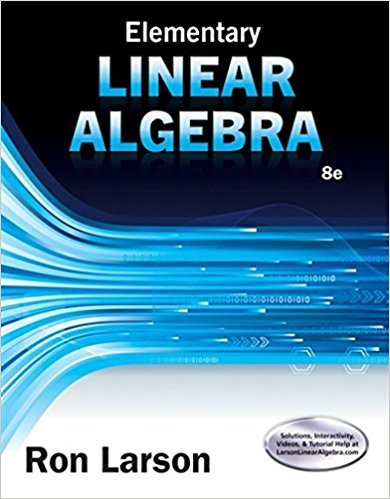×
×

# Solutions for Chapter 3: Determinants## Full solutions for Elementary Linear Algebra | 8th Edition

ISBN: 9781305658004Solutions for Chapter 3: Determinants

Solutions for Chapter 3
4 5 0 300 Reviews
16
4
##### ISBN: 9781305658004

Elementary Linear Algebra was written by and is associated to the ISBN: 9781305658004. Since 156 problems in chapter 3: Determinants have been answered, more than 45025 students have viewed full step-by-step solutions from this chapter. This textbook survival guide was created for the textbook: Elementary Linear Algebra, edition: 8. This expansive textbook survival guide covers the following chapters and their solutions. Chapter 3: Determinants includes 156 full step-by-step solutions.

Key Math Terms and definitions covered in this textbook
• Block matrix.

A matrix can be partitioned into matrix blocks, by cuts between rows and/or between columns. Block multiplication ofAB is allowed if the block shapes permit.

• Column picture of Ax = b.

The vector b becomes a combination of the columns of A. The system is solvable only when b is in the column space C (A).

• Column space C (A) =

space of all combinations of the columns of A.

• Commuting matrices AB = BA.

If diagonalizable, they share n eigenvectors.

A sequence of steps (end of Chapter 9) to solve positive definite Ax = b by minimizing !x T Ax - x Tb over growing Krylov subspaces.

• Diagonalizable matrix A.

Must have n independent eigenvectors (in the columns of S; automatic with n different eigenvalues). Then S-I AS = A = eigenvalue matrix.

• Gauss-Jordan method.

Invert A by row operations on [A I] to reach [I A-I].

• Hilbert matrix hilb(n).

Entries HU = 1/(i + j -1) = Jd X i- 1 xj-1dx. Positive definite but extremely small Amin and large condition number: H is ill-conditioned.

• Krylov subspace Kj(A, b).

The subspace spanned by b, Ab, ... , Aj-Ib. Numerical methods approximate A -I b by x j with residual b - Ax j in this subspace. A good basis for K j requires only multiplication by A at each step.

• lA-II = l/lAI and IATI = IAI.

The big formula for det(A) has a sum of n! terms, the cofactor formula uses determinants of size n - 1, volume of box = I det( A) I.

• Partial pivoting.

In each column, choose the largest available pivot to control roundoff; all multipliers have leij I < 1. See condition number.

• Rank one matrix A = uvT f=. O.

Column and row spaces = lines cu and cv.

• Rank r (A)

= number of pivots = dimension of column space = dimension of row space.

• Row picture of Ax = b.

Each equation gives a plane in Rn; the planes intersect at x.

• Schur complement S, D - C A -} B.

Appears in block elimination on [~ g ].

• Spanning set.

Combinations of VI, ... ,Vm fill the space. The columns of A span C (A)!

• Spectrum of A = the set of eigenvalues {A I, ... , An}.

Spectral radius = max of IAi I.

• Toeplitz matrix.

Constant down each diagonal = time-invariant (shift-invariant) filter.

• Trace of A

= sum of diagonal entries = sum of eigenvalues of A. Tr AB = Tr BA.

• Vector v in Rn.

Sequence of n real numbers v = (VI, ... , Vn) = point in Rn.

×